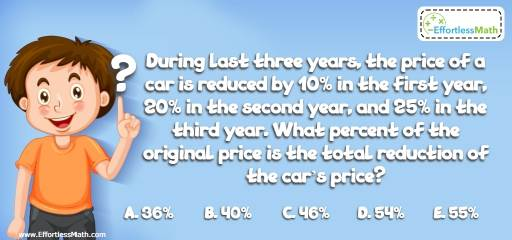# Ratio, Proportion and Percentages Puzzle – Challenge 28

This is a great math and critical thinking challenge to strengthen a child's grasp of math concepts in a fun way.## Challenge:

During the last three years, the price of a car has been reduced by $$10\%$$ in the first year, $$20\%$$ in the second year, and $$25\%$$ in the third year. What percent of the original price is the total reduction of the car’s price?

A- $$36\%$$

B- $$40\%$$

C- $$46\%$$

D- $$54\%$$

E- $$55\%$$

### The Absolute Best Book to Challenge Your Smart Student!

Choose x for current price of the car. In the first year, the price of the car reduced to $$0.90 x$$. Next year, it’ll be
$$0.90x × 0.80 = 0.72x$$.
For the third year, the price of the car is: $$0.72x × 0.75 = 0.54 x$$
Therefore, $$46\%$$ of the original price is the total reduction of the car’s price.

The Absolute Best Books to Ace Algebra

### What people say about "Ratio, Proportion and Percentages Puzzle – Challenge 28 - Effortless Math: We Help Students Learn to LOVE Mathematics"?

No one replied yet.

X
45% OFF

Limited time only!

Save Over 45%

SAVE $40 It was$89.99 now it is \$49.99